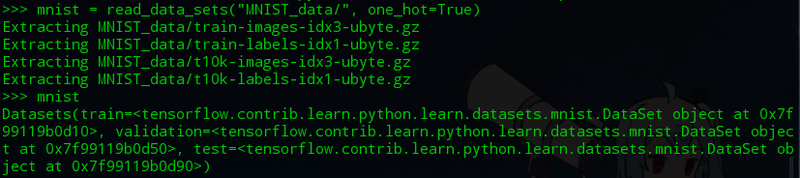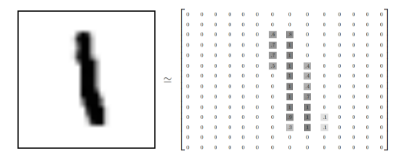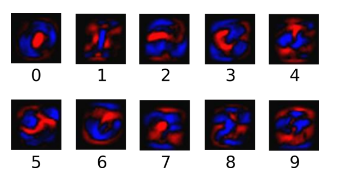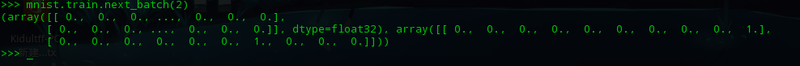# TensorFlow MNIST机器学习入门详解

0x00、何为MNIST？

0x01、准备

• 训练所用图片（总共60000张）

• 训练所用标签（解释了图片的内容）

• 测试所用图片（总共10000张

• 测试所用标签（测试图片的正确答案）

``````from __future__ import absolute_import
from __future__ import division
from __future__ import print_function
import gzip
import os
import tempfile
import numpy
from six.moves import urllib
from six.moves import xrange  # pylint: disable=redefined-builtin
import tensorflow as tf

0x02、数据集

``mnist = read_data_sets("MNIST_data/", one_hot=True)``导入的数据（训练所用的60000份数据集，记做mnist.train，和测试所用的10000份，记做mnist.test。其中所有的图片记做xs，标签记做ys。）在标签集中，mnist.train.labels（训练标签集）是一个形状为[60000, 10]的张量，第一个依然是索引，第二个表示标签表示的第几个数字。例如 0表示为[1,0,0,0,0,0,0,0,0,0]

好了，接下来我们可以构建模型了

0x03、Softmax回归

3            8softmax函数即可将证据值转化为概率。0x04、TensorFlow实现模型

``x = tf.placeholder("float", [None, 784])``

``````W = tf.Variable(tf.zeros([784,10]))
b = tf.Variable(tf.zeros())``````

W 的维度是[784，10]，因为我们想要用784维的图片向量乘以它以得到一个10维的证据值向量，每一位对应不同数字类。b 的形状是，所以我们可以直接把它加到输出上面。

``y = tf.nn.softmax(tf.matmul(x,W) + b)``

tf.matmul(X，W) 表示 x 乘以 W，对应上面那个式子中的 Wx。

b是偏置量，对应式子中的bi。

0x05、TensorFlow训练

y 是我们预测的概率分布, y' 是实际的分布。

``y_ = tf.placeholder("float", [None,10])````cross_entropy = -tf.reduce_sum(y_*tf.log(y))``

``train_step = tf.train.GradientDescentOptimizer(0.01).minimize(cross_entropy)``

``init = tf.initialize_all_variables()``

``````sess = tf.Session()
sess.run(init)``````

``````for i in range(1000):
batch_xs, batch_ys = mnist.train.next_batch(100)
sess.run(train_step, feed_dict={x: batch_xs, y_: batch_ys})``````

array( array(表示图片像素的784维向量, 表示图片对应的数字的10维向量)， array(像素的784维向量, 数字的10维向量) ...... )0x06、评估

``correct_prediction = tf.equal(tf.argmax(y,1), tf.argmax(y_,1))``

``accuracy = tf.reduce_mean(tf.cast(correct_prediction, "float"))``

``print sess.run(accuracy, feed_dict={x: mnist.test.images, y_: mnist.test.labels})``

0x07、总结

``````# -*- coding:utf-8 -*-
from __future__ import absolute_import
from __future__ import division
from __future__ import print_function
import gzip
import os
import tempfile
import numpy
from six.moves import urllib
from six.moves import xrange  # pylint: disable=redefined-builtin
import tensorflow as tf
################# 搭建模型 #################
x = tf.placeholder("float", [None, 784])  #用来表示图像形状的占位符
W = tf.Variable(tf.zeros([784,10]))          #权重值
b = tf.Variable(tf.zeros())      #偏置率
evidence = tf.matmul(x,W) + b      #证据=累加(W*x+b)
y = tf.nn.softmax(evidence)      #y表示概率。matmul表示相乘。x与W相乘，加上偏置率
################# 训练模型 #################
y_ = tf.placeholder("float", [None,10])    #用来表示交叉熵的占位符
cross_entropy = -tf.reduce_sum(y_*tf.log(y))#交叉熵=-累加(y_*log(y))
#0.01的学习速率最小化交叉熵
init = tf.initialize_all_variables()    #初始化所有变量
sess = tf.Session()
sess.run(init)          #启动会话
for i in range(1000):              #学习1000次
batch_xs, batch_ys = mnist.train.next_batch(100)
#batch_xs为图像像素向量，batch_ys为标签向量
sess.run(train_step, feed_dict={x: batch_xs, y_: batch_ys})
#运行会话。按照train_step的方式，将像素和标签传入，进行学习
################# 评估好坏 #################
correct_prediction = tf.equal(tf.argmax(y,1), tf.argmax(y_,1))
#判断是否相等(是否正确)
accuracy = tf.reduce_mean(tf.cast(correct_prediction, "float"))
#reduce_mean用来求平均值。cast用来布尔转浮点(true=>1)
print(sess.run(accuracy, feed_dict={x: mnist.test.images, y_: mnist.test.labels}))
#用所有测试图片和标签进行测试。``````

运行后准确率大致是91%。